# FUNDAMENTAL GROUP OF TORUS HOMOTOPY FUNDAMENTAL GROUP OF

• Slides: 10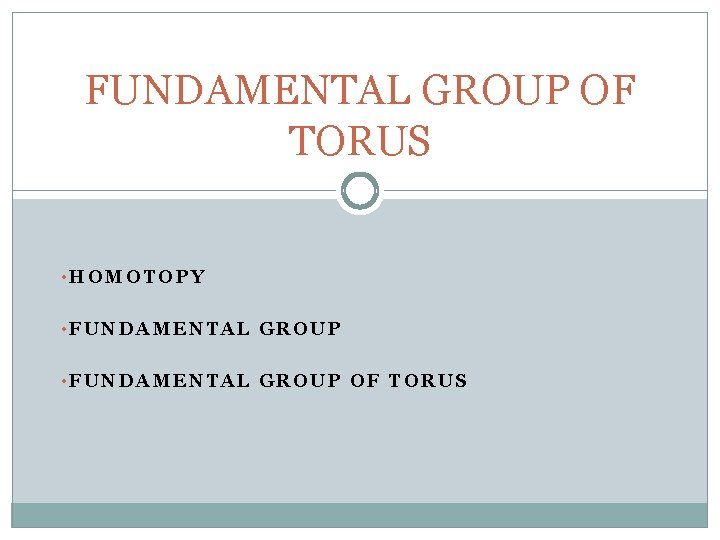FUNDAMENTAL GROUP OF TORUS • HOMOTOPY • FUNDAMENTAL GROUP OF TORUSHOMOTOPY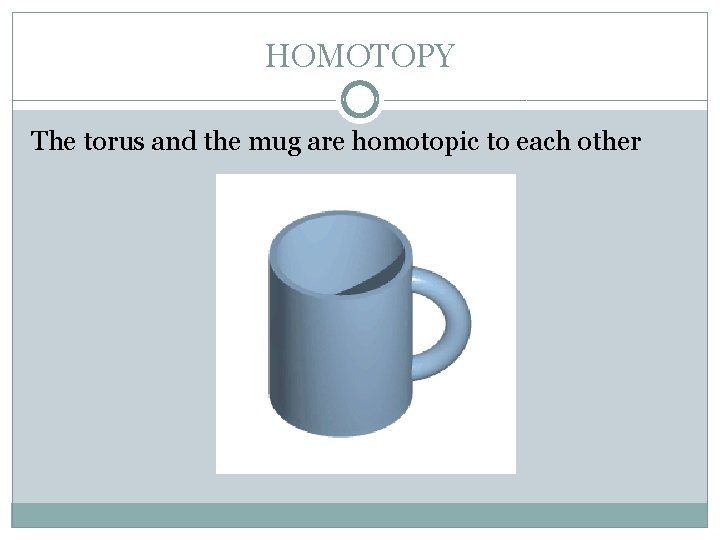HOMOTOPY The torus and the mug are homotopic to each other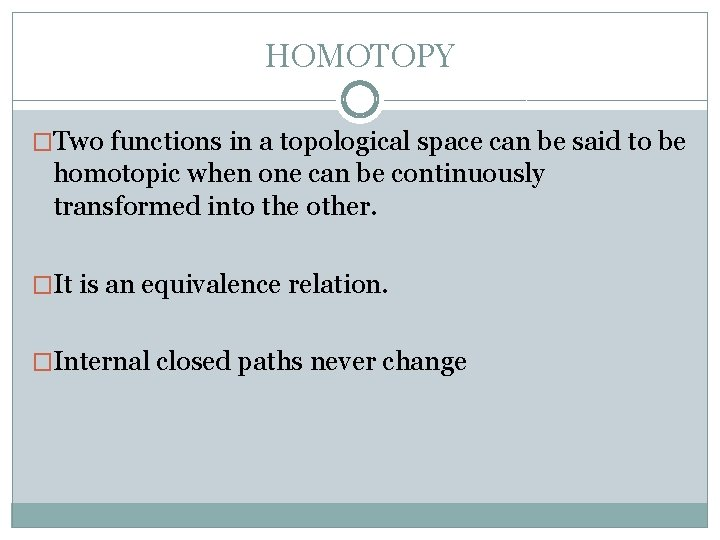HOMOTOPY �Two functions in a topological space can be said to be homotopic when one can be continuously transformed into the other. �It is an equivalence relation. �Internal closed paths never change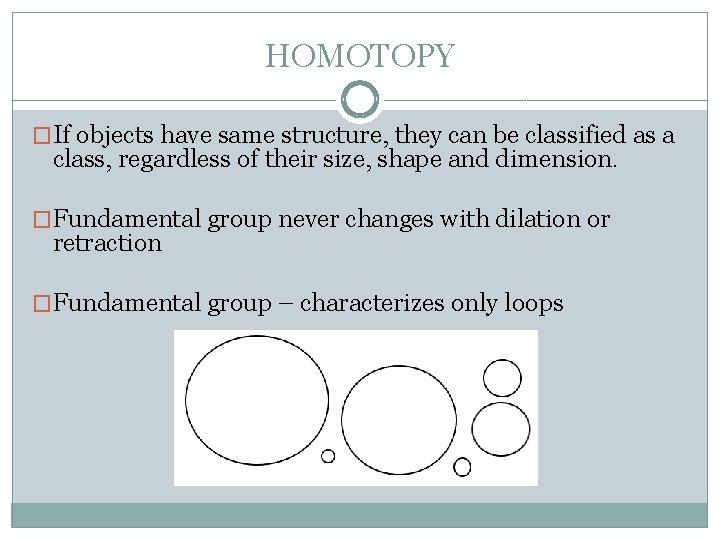HOMOTOPY �If objects have same structure, they can be classified as a class, regardless of their size, shape and dimension. �Fundamental group never changes with dilation or retraction �Fundamental group – characterizes only loops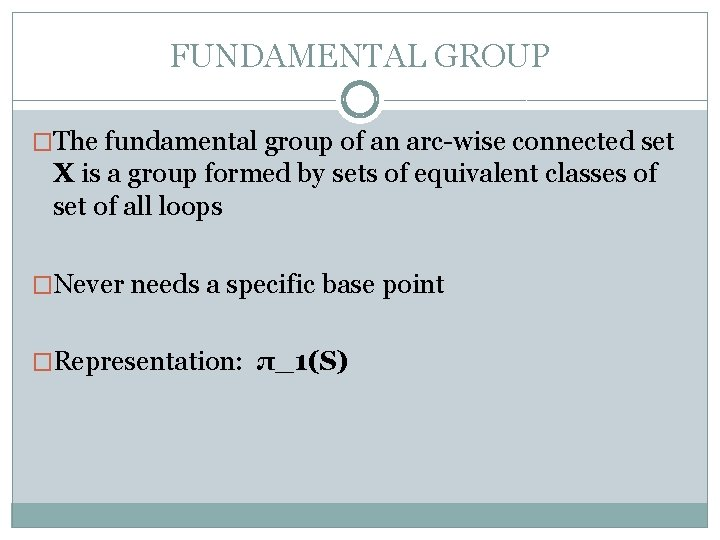FUNDAMENTAL GROUP �The fundamental group of an arc-wise connected set X is a group formed by sets of equivalent classes of set of all loops �Never needs a specific base point �Representation: π_1(S)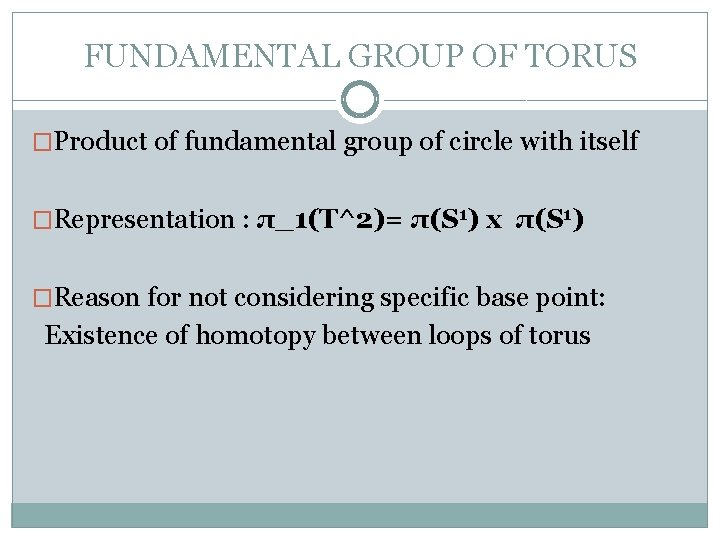FUNDAMENTAL GROUP OF TORUS �Product of fundamental group of circle with itself �Representation : π_1(T^2)= π(S 1) x π(S 1) �Reason for not considering specific base point: Existence of homotopy between loops of torusFUNDAMENTAL GROUP OF TORUS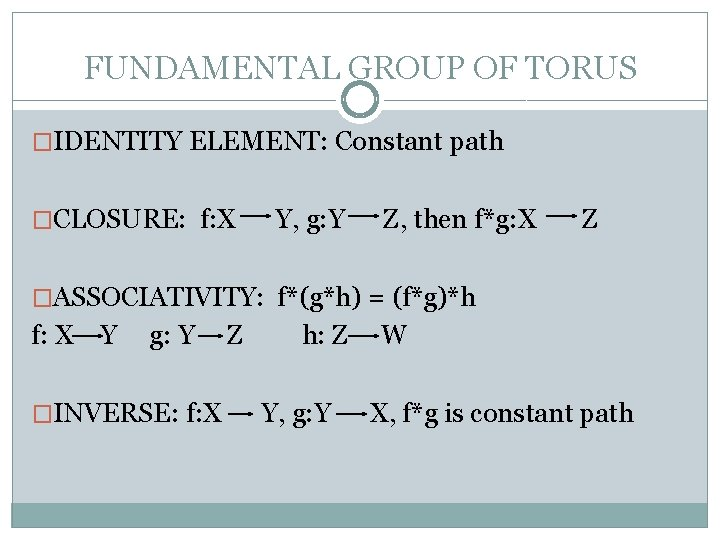FUNDAMENTAL GROUP OF TORUS �IDENTITY ELEMENT: Constant path �CLOSURE: f: X Y, g: Y Z, then f*g: X Z �ASSOCIATIVITY: f*(g*h) = (f*g)*h f: X Y g: Y �INVERSE: f: X Z h: Z Y, g: Y W X, f*g is constant pathTHANK YOU